# Linear programming duality

The conventional statement of linear programming duality is completely inscrutable.

• Prime: maximise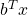subject to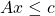and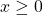.
• Dual: minimise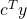subject to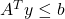and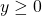.

If either problem has a finite optimum then so does the other, and the optima agree.

do understand concrete examples.  Suppose we want to pack the maximum number vertex-disjoint copies of a graphinto a graph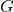.  In the fractional relaxation, we want to assign each copy ofa weight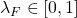such that the weight of all the copies ofat each vertex is at most, and the total weight is as large as possible.  Formally, we want to

maximise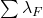subject to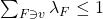and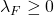,

which dualises to

minimise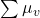subject to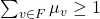and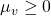.

That is, we want to weight vertices as cheaply as possible so that every copy ofcontains(fractional) vertex.

To get from the prime to the dual, all we had to was change a max to a min, swap the variables indexed byfor variables indexed by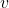and flip one inequality.  This is so easy that I never get it wrong when I’m writing on paper or a board!  But I thought for years that I didn’t understand linear programming duality.

(There are some features of this problem that make things particularly easy: the vectors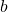and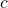in the conventional statement both have all their entries equal to, and the matrix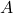is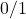-valued.  This is very often the case for problems coming from combinatorics.  It also matters that I chose not to make explicit that the inequalities should hold for every(or, as appropriate).)

Returning to the general statement, I think I’d be happier with

• Prime: maximise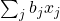subject to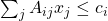and.
• Dual: minimise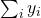subject to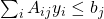and.

My real objection might be to matrix transposes and a tendency to use notation for matrix multiplication just because it’s there.  In this setting a matrix is just a function that takes arguments of two different types (andor, if you must,and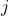), and I’d rather label the types explicitly than rely on an arbitrary convention.